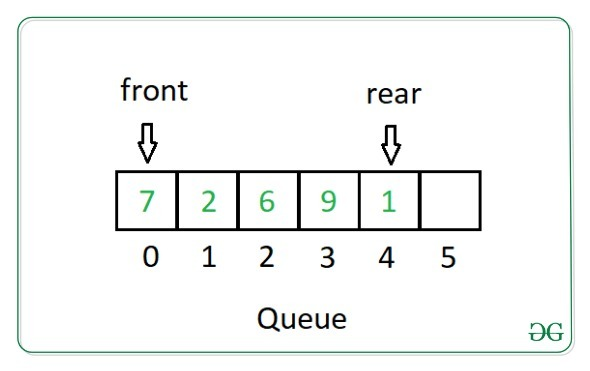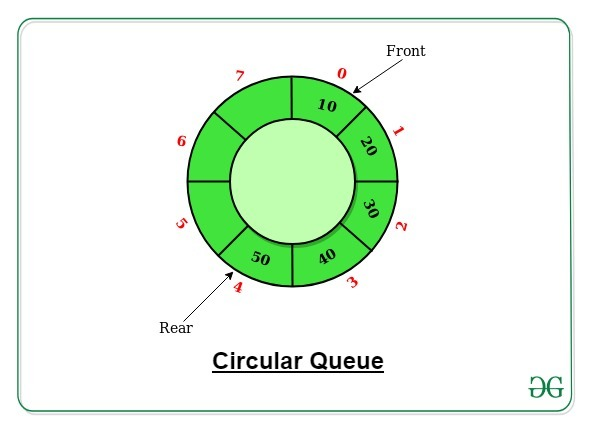# Perl | Implementing a Queue

• Last Updated : 01 Sep, 2021

Prerequisite: Stack
Queue in Perl is a linear abstract data structure which follows the FIFO (First In First Out) order. It resembles the properties of the queue which we encounter in our daily life where the person who comes first will be served first. It is open at both ends. Unlike stack where the operation of insertion and deletion occurs at same end called the top of stack, in queue these operations occur at different ends called the front and rear end of the queue.

### Operations on Queue:

The following basic operations are performed on a queue:

1. Enqueue (Insertion): Adds an item to the queue. An Overflow condition occurs if the queue is full.
2. Dequeue (deletion): Removes an item from the queue. An Underflow condition occurs if the queue is empty.
3. Front: Get the front item from queue.
4. Rear: Get the last item from queue.### Creating a Queue

Creating a queue in Perl is very simple. It can be done by declaring an array which can be either empty or initialized with some pre-filled data.

```@queue;        # Queue is empty.

@queue = (1, 2, 3);      # Queue with initialized values.```

### Types of Queues

Based on the criterion of Insertion and deletion, queues can be of the following types:

### Simple Queue

Simple Queue is simply a queue where insertion takes place at front and deletion takes place at end of the queue.
In Perl, the operations of a queue can be implemented using push and shift functions.
The push function is used to add one or more values at the end of the queue.
The shift function will move the queue one unit to the left deleting (popping) the first element. It will return undef in case of empty queue.

Examples:

## Perl

 `#!/usr/bin/perl``use` `strict;``use` `warnings;``  ` `# Intitialising the Queue``my` `@queue` `= (1, 2, 3);``  ` `# Original queue``print` `"Original Queue: @queue"``;` `# Pushing values into queue``push``(``@queue``, (4, 5, 6)); ``  ` `# Updated Queue after ``# Push operation``print``(``"\nUpdated Queue after Push: @queue"``);`  `# Popping values from queue``my` `\$val1` `= ``shift``(``@queue``);``print``(``"\nElement popped is: \$val1"``);``my` `\$val2` `= ``shift``(``@queue``);``print``(``"\nElement popped is: \$val2"``); ``  ` `# Updated Queue after ``# Pop operations``print``(``"\nUpdated Queue after Pop: @queue"``);`
Output:
```Original Queue: 1 2 3
Updated Queue after Push: 1 2 3 4 5 6
Element popped is: 1
Element popped is: 2
Updated Queue after Pop: 3 4 5 6```

### Circular Queue

In circular queue, the last position of the queue is connected to the first position to make a circle, also called as Ring Buffer. If the queue is empty from front side but full at the end, then also we can insert elements in the circular queue, which is not the case in the simple queue. The circular queue will be of fixed size, unlike a normal queue.
In additions to push and shift functions, circular queue also has two variables front and rear to store the front and the rear positions of the queue.Example:

## Perl

 `#!/usr/bin/perl``use` `strict;``use` `warnings;` `# Intitialising the Queue``my` `@queue` `= (1, 2, 3);``my` `\$size` `= 7; ``# No. of elements to be stored in queue` `# Initially queue has three elements``my` `\$front` `= 0;``my` `\$rear` `= 2;` `# Original queue``print` `"Original Queue: @queue"``;` `# Pushing values into queue``\$rear` `= (``\$rear` `+ 1) % ``\$size``;``my` `\$val` `= 4;``while``(1)``{``    ``if` `(``\$rear` `== ``\$front``)``    ``{``        ``print``(``"\nQueue is full."``);``        ``last``;``    ``}``    ``else``    ``{``        ``print``(``"\nPushed \$val in queue"``);``        ``\$queue``[``\$rear``] = ``\$val``;``        ``\$rear` `= (``\$rear` `+ 1) % ``\$size``;``        ``\$val` `+= 1;``    ``}``}` `# Updated Queue after``# Push operation``print``(``"\nUpdated Queue after Push: @queue"``);` `# Popping values from queue``my` `\$val1` `= ``\$queue``[``\$front``];``\$queue``[``\$front``] = -1;``\$front` `= (``\$front` `+ 1) % ``\$size``;``print``(``"\nElement popped is: \$val1"``);` `my` `\$val2` `= ``\$queue``[``\$front``];``\$queue``[``\$front``] = -1;``\$front` `= (``\$front` `+ 1) % ``\$size``;``print``(``"\nElement popped is: \$val2"``);` `# Updated Queue after``# Pop operations``print``(``"\nUpdated Queue after Pop: @queue"``);``while``(1)``{``    ``if` `(``\$rear` `% ``\$size` `== ``\$front``)``    ``{``        ``print``(``"\nQueue is full."``);``        ``last``;``    ``}``    ``else``    ``{``        ``print``(``"\nPushed \$val in queue"``);``        ``\$queue``[``\$rear``] = ``\$val``;``        ``\$rear` `+= 1;``        ``\$val` `+= 1;``    ``}``}``print``(``"\nUpdated Queue after Push: @queue"``);`
Output:
```Original Queue: 1 2 3
Pushed 4 in queue
Pushed 5 in queue
Pushed 6 in queue
Pushed 7 in queue
Queue is full.
Updated Queue after Push: 1 2 3 4 5 6 7
Element popped is: 1
Element popped is: 2
Updated Queue after Pop: -1 -1 3 4 5 6 7
Pushed 8 in queue
Pushed 9 in queue
Queue is full.
Updated Queue after Push: 8 9 3 4 5 6 7```

### Priority Queue

In priority queue, every item has a defined priority associated with it. An item having the least priority will be the first to be removed from the priority queue. The items with same priority will be dealt with in the order of their
position in the queue.

Examples:

## Perl

 `#!/usr/bin/perl``use` `strict;``use` `warnings;` `# Initialising queue with priority``my` `@queue` `= ([1, 3], [2, 7], [4, 3], [5, 2]);` `sub` `pull_highest_prio``{``    ``my` `\$max` `= 0;``    ``for` `(``my` `\$i` `= 0; ``\$i` `< ``scalar``(``@queue``); ``\$i``++)``    ``{``        ``if` `(``\$queue``[``\$i``] > ``\$queue``[``\$max``])``        ``{``            ``\$max` `= ``\$i``;``        ``}``    ``}``    ``print``(``"\nPopped item: \$queue[\$max], "``,``          ``"Priority: \$queue[\$max]"``);``    ``splice` `@queue``, ``\$max``, 1;``}` `# Driver Code``print``(``"\nOriginal Queue is "``);``for` `(``my` `\$i` `= 0; ``\$i` `< 4; ``\$i``++)``{``    ``print``(``"\$queue[\$i] "``);``}``push``(``@queue``, [11, 9]);``push``(``@queue``, [7, 0]);` `print``(``"\nUpdated Queue after push is "``);``for` `(``my` `\$i` `= 0; ``\$i` `< 6; ``\$i``++)``{``    ``print``(``"\$queue[\$i] "``);``}``pull_highest_prio;``pull_highest_prio;``pull_highest_prio;``pull_highest_prio;` `print``(``"\nUpdated Queue after pop is "``);``for` `(``my` `\$i` `= 0; ``\$i` `< 2; ``\$i``++)``{``    ``print``(``"\$queue[\$i] "``);``}`
Output:
```Original Queue is 1 2 4 5
Updated Queue after push is 1 2 4 5 11 7
Popped item: 11, Priority: 9
Popped item: 2, Priority: 7
Popped item: 1, Priority: 3
Popped item: 4, Priority: 3
Updated Queue after pop is 5 7```

### Deque(Doubly Ended Queue)

Doubly Ended Queue is just a generalized version of simple queue, except the insertion and deletion operations, can be done at both Front and Rear end of the queue. It is a very important type of data structure as it can be used both as a stack or a queue.

Examples:

## Perl

 `#!/usr/bin/perl``use` `strict;``use` `warnings;` `# Intitialising the Queue``my` `@queue` `= (1, 2, 3);``my` `\$front` `= 0;``my` `\$rear` `= 2;``sub` `insert_front``{``    ``my` `\$val` `= ``\$_``;``    ``unshift``(``@queue``, ``\$val``);``    ``\$rear` `+= 1;``}` `sub` `insert_rear``{``    ``my` `\$val` `= ``\$_``;``    ``push``(``@queue``, ``\$val``);``    ``\$rear` `+= 1;``}` `sub` `delete_front``{``    ``print``(``"\nElement popped is: \$queue"``);``    ``splice` `@queue``, 0, 1;``    ``\$rear` `-= 1;``}` `sub` `delete_rear``{``    ``print``(``"\nElement popped is: \$queue[\$rear]"``);``    ``splice` `@queue``, ``scalar``(``@queue``) - 1, 1;``    ``\$rear` `-= 1;``}` `# Original queue``print` `"Original Queue: @queue"``;` `# Pushing values into queue``&insert_rear(4);``&insert_rear(3);``print``(``"\nRear element is \$queue[\$rear]"``);``&insert_front(1);``&insert_front(7);``print``(``"\nFront element is \$queue[\$front]"``);` `# Updated Queue after``# Push operation``print``(``"\nUpdated Queue after Push: @queue"``);` `# Popping values from queue``delete_rear;``delete_front;``print``(``"\nFront element is \$queue[\$front]"``);` `&insert_front(20);``delete_rear;``print``(``"\nRear element is \$queue[\$rear]"``);` `# Updated Queue after``# Pop operations``print``(``"\nUpdated Queue after Pop: @queue"``);`
Output:
```Original Queue: 1 2 3
Rear element is 3
Front element is 7
Updated Queue after Push: 7 1 1 2 3 4 3
Element popped is: 3
Element popped is: 7
Front element is 1
Element popped is: 4
Rear element is 3
Updated Queue after Pop: 20 1 1 2 3```

### Abstract Implementation

Abstract means to hide the functionality of the operations from the normal user, that is to provide the operational functionality without defining the logic behind the function.
In Perl, the abstract implementation of queue can be achieved using Perl’s in-built module Thread::Queue which provide thread-safe FIFO queues that can be accessed by any number of threads.

Basic methods:

1. new(list) – Creates a new queue with the provided list of items or if not listed it creates a new empty queue.
2. enqueue(list) – Adds a list of items onto the end of the queue.
3. dequeue(COUNT) – Removes the requested number of items from the head of the queue, and returns them and if not mentioned it dequeues one element from the queue.
4. pending() – Returns the number of items still left in the queue or we can say, it basically returns the size of the queue.
5. end() – declares that no more items will be added to the queue.

Examples:

## Perl

 `#!/usr/bin/perl``use` `strict;``use` `warnings;` `use` `Thread::Queue;` `# A new pre-populated queue``my` `\$q` `= Thread::Queue->new(1, 2, 3);    ` `sub` `head``{``    ``if` `(``\$q``->pending() == 0)``    ``{``        ``print``(``"\nThe queue is empty."``);``    ``}``    ``else``    ``{``        ``my` `\$item` `= ``\$q``->dequeue();``        ``print``(``"\nHead of the Queue is \$item."``);``    ``}``}``my` `\$isize` `= ``\$q``->pending();``print``(``"Initial size of the queue is \$isize"``);` `# insert three items at the back of the queue``\$q``->enqueue(5, 6, 7);    ``&head();` `my` `\$size` `= ``\$q``->pending();``print``(``"\nThe size of the queue is \$size"``);` `# delete the head item of the queue``\$q``->dequeue();            ``&head();` `# delete two items from the front``\$q``->dequeue(2);        ``my` `\$size2` `= ``\$q``->pending();``print``"\nThe size of the queue is \$size2"``;` `&head();``&head();``\$q``->end();`
Output:
``` size of the queue is 3
Head of the Queue is 1.
The size of the queue is 5
Head of the Queue is 3.
The size of the queue is 1
Head of the Queue is 7.
The queue is empty.```

### Parallel or Asynchronous Processing

The word Parallel means simultaneously or at the same time and Asynchronous refers to continuous or non-blocking. The implementation operations of a queue are blocking i.e while performing one operation the other operation have to wait for it to end first and are then executed. This is fine for small number of tasks but for a large number of tasks or operations, this type of processing is a bit hectic and time-consuming.
To overcome this problem, Perl provide some way of doing parallel or asynchronous processing so that these operations can run simultaneously or without waiting for other to end. The two major solutions for this is threading and forking. Forking is to create exactly same copy of some process and then these two processes executes in parallel without much connection between their variables, although they manipulate or work upon same variables(copy). It is done using fork() function.

Examples:

## Perl

 `#!/usr/bin/perl``use` `strict;``use` `warnings;``use` `5.010;``     ` `say` `"PID \$\$"``;``my` `\$pid` `= ``fork``();``die` `if` `not ``defined` `\$pid``;``say` `"PID \$\$ (\$pid)"``;`
Output:
```PID 22112
PID 22112 (22113)
PID 22113 (0)```

Before calling fork(), we got a PID (22112) which is the process ID for current process, after forking we got two lines. The first printed line came from the same process as the current (it has the same PID), the second printed line came from the child process (with PID 63779). The first one received a \$pid from fork containing the number of the child process. The second, the child process got the number 0.
Perl also supports event-driven programming through POE framework.

My Personal Notes arrow_drop_up